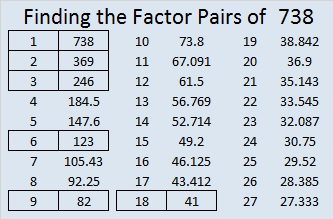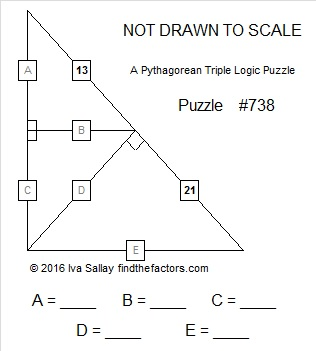# 738 Warning: These 3 right triangles do NOT form one large right triangle

• 738 is a composite number.
• Prime factorization: 738 = 2 x 3 x 3 x 41, which can be written 738 = 2 x 3² x 41
• The exponents in the prime factorization are 1, 2, and 1. Adding one to each and multiplying we get (1 + 1)(2 + 1)(1 + 1) = 2 x 3 x 2 = 12. Therefore 738 has exactly 12 factors.
• Factors of 738: 1, 2, 3, 6, 9, 18, 41, 82, 123, 246, 369, 738
• Factor pairs: 738 = 1 x 738, 2 x 369, 3 x 246, 6 x 123, 9 x 82, or 18 x 41
• Taking the factor pair with the largest square number factor, we get √738 = (√9)(√82) = 2√82 ≈ 27.166155.The puzzle below is NOT drawn to scale. Angles that are marked as right angles are 90 degrees, but any angle that looks like a 45 degree angle, isn’t 45 degrees. Lines that look parallel are NOT parallel. Shorter looking line segments may actually be longer than longer looking line segments. Most rules of geometry do not apply here: in fact non-adjacent triangles in the drawing might actually overlap.

No geometry is needed to solve this puzzle. All that is needed is the table of Pythagorean triples under the puzzle. The puzzle only uses triples in which each leg and each hypotenuse is less than 100 units long. The puzzle has only one solution.

Go ahead, give it a try!Print the puzzles or type the solution on this excel file: 12 Factors 2016-01-11Some time ago I published a rather ambitious Pythagorean triple logic puzzle. I didn’t post the answers but invited anyone who desired to post some or all of the answers and to do so in the comments. As of today, no one has posted any answers. Perhaps that puzzle was too difficult. I decided to post a SIMPLER one today. Just follow the instructions above the puzzle.

———————————————————

Now for some facts about the number 738:

Because 41 is one of its factors, 738 is the hypotenuse of Pythagorean triple 162-720-738. What is the greatest common factor of the three numbers in the triple? It’s the other number in the same factor pair as 41.

738 can be expressed as the sum of consecutive numbers four different ways:

• 245 + 246 + 247 = 738; that’s 3 consecutive numbers.
• 78 + 79 + 80 + 81 + 82 + 83 + 84 + 85 + 86 = 738; that’s 9 consecutive numbers.
• 56 + 57 + 58 + 59 + 60 + 61 + 62 + 63 + 64 + 65 + 66 + 67 = 738; that’s 12 consecutive numbers.
• 3 + 4 + 5 + 6 + 7 + 8 + 9 + 10 + 11 + 12 + 13 + 14 + 15 + 16 + 17 + 18 + 19 + 20 + 21 + 22 + 23 + 24 + 25 + 26 + 27 + 28 + 29 + 30 + 31 + 32 + 33 + 34 + 35 + 36 + 37 + 38; that’s 36 consecutive numbers.

738 is a palindrome in base 15 and base 16, two consecutive bases:

• 343 BASE 15; note that 3(225) + 4(15) + 3(1) = 738.
• 2E2 BASE 16 (E = 14 base 10); note that 2(256) + 14(16) + 2(1) = 738.

From OEIS.org I learned that 6 + 66 + 666 = 738. Cool! Six 6’s were used!

———————————————————

## 2 thoughts on “738 Warning: These 3 right triangles do NOT form one large right triangle”

1.nerdinthebrain

I think I have the answer, but I don’t want to leave a spoiler comment. 😀 Now I’m off to check out the other one you mentioned…somehow I managed to totally miss it when you posted it!

•ivasallay